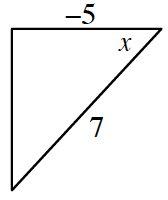### Home > PC3 > Chapter 9 > Lesson 9.2.2 > Problem9-119

9-119.

Suppose $\cos(x)=-\frac { 5 } { 7 }$ where $π < x <\frac { 3 \pi } { 2 }$.

1. Determine the exact values of the five other trigonometric ratios. Rationalize the denominator if necessary.

Sketch a diagram of this situation.Now calculate the length of the missing leg.
Then you should be able to state the all of the trigonometric ratios.

2. Calculate the exact values of $\sin(2x)$ and $\cos(2x)$.

$\sin(2x) = 2\sin(x)\cos(x)\\ \cos(2x) = \cos2(x) − \sin2(x)$